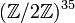# Group cohomology of elementary abelian group:E16

## Contents

View group cohomology of particular groups | View other specific information about elementary abelian group:E16

## Family contexts

Family Parameter values Information on group cohomology of family
elementary abelian group of order$p^n$ for a prime$p$$p = 2, n = 4$ group cohomology of elementary abelian groups
elementary abelian group of prime-fourth order for a prime$p$$p = 2$ group cohomology of elementary abelian group of prime-fourth order

## Homology groups for trivial group action

FACTS TO CHECK AGAINST (homology group for trivial group action):
First homology group: first homology group for trivial group action equals tensor product with abelianization
Second homology group: formula for second homology group for trivial group action in terms of Schur multiplier and abelianization|Hopf's formula for Schur multiplier
General: universal coefficients theorem for group homology|homology group for trivial group action commutes with direct product in second coordinate|Kunneth formula for group homology

### Over the integers=

The homology groups over the integers are given as follows:$\! H_q(E_{16};\mathbb{Z}) = \left\lbrace\begin{array}{rl} (\mathbb{Z}/2\mathbb{Z})^{\frac{2q^3 + 15q^2 + 34q + 45}{24}}, & \qquad q = 1,3,5,\dots \\ (\mathbb{Z}/2\mathbb{Z})^{\frac{2q^3 + 15q^2 + 34q}{24}}, & \qquad q = 2,4,6,\dots \\ \mathbb{Z}, & \qquad q = 0 \\\end{array}\right.$

The first few homology groups are given as follows:$q$$0$$1$$2$$3$$4$$5$$6$$7$$8$$H_q$$\mathbb{Z}$$(\mathbb{Z}/2\mathbb{Z})^4$$(\mathbb{Z}/2\mathbb{Z})^6$$(\mathbb{Z}/2\mathbb{Z})^{14}$$(\mathbb{Z}/2\mathbb{Z})^{21}$$(\mathbb{Z}/2\mathbb{Z})^{35}$$(\mathbb{Z}/2\mathbb{Z})^{49}$$(\mathbb{Z}/2\mathbb{Z})^{71}$$(\mathbb{Z}/2\mathbb{Z})^{94}$
rank of$H_q$ as an elementary abelian 2-group -- 4 6 14 21 35 49 71 94